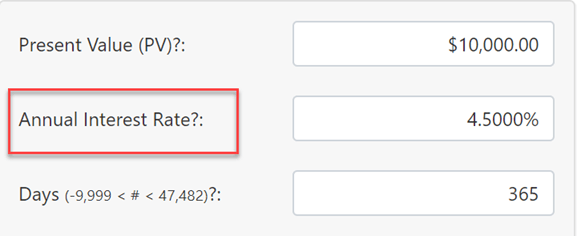# Future Value CalculatorFuture value of money as of any date.

advertisement

The value of money will change over time. Meaning, what a dollar will buy today is not what a dollar will buy in the future. What the dollar buys in the future is called its future value. A future value calculator is the tool one uses to calculate a dollar's future value.

Two factors impact the dollar's FV (or any currency's FV):

• inflation (or deflation)
• investment return

The greater the rate of inflation the less the dollar will buy. The greater the investment's rate-of-return (or interest rate) or the greater the rate of deflation, the more the dollar will buy.

This future value calculator will calculate the FV of an amount or asset after an exact number of days assuming any rate-of-return (tested to 99% per annum) for 12 compounding frequencies plus simple interest.

Because this calculator is date sensitive, and because it supports many compounding options, it is a suitable tool for calculating the balance of a debt when the debtor has not made any payments. More details below the calculator

×

#### Info...

Original Size

Copy and paste this URL to save your inputs to share them with others.

Click "Calc", "Clear", "Preview." or "Schedule" to update the URL. Paste it into any browser to reload.

## More About Future Value

The future value calculator normally calculates a nominal future value. This means the calculated future value is the result of an investment gain or from interest earned on the money. A nominal future value does not account for inflation.

If you want to know the real future value, you can do one of two things.

advertisement

If you want to to know the purchasing power of the original amount after inflation, then deduct an estimated inflation rate from the Annual Interest Rate. Example: If your Annual Interest Rate is 4.5%, and you estimate that inflation will average 2% per year, then rather than enter 4% for your rate-of-return, enter 2.5% instead. The calculated FV will now be the real future value.In this case, for our purposes, interest rate and rate-of-return are the same thing.

This is a bit of an oxymoron more though. Economists call this the real future value, but it's actually an estimated real future value because we can only estimate the future rate of inflation.

Nonetheless, the real future value is closer to an accurate calculation than the nominal future value which as you can see, doesn't even consider inflation.

If on the other hand, you want to be compensated for inflation, then you add the inflation rate to the annual interest rate. If for example, someone had owed you \$2,000 for five and a half years and they agreed to compensate you for 2% inflation on top of a 4% interest rate, then you would add the inflation rate to the rate of return.

The result thus includes the effect of inflation. Or you can think of the calculation this way. If I want to make a real 4.5% gain on my investment, how much will I need to also compensate for the loss of value due to inflation?

advertisement

## 12 Comments on “Future Value Calculator”

Join the conversation. Tell me what you think.

advertisement